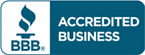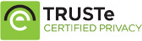# Solution manual for Statistics for Business and Economics 11th Edition by David R. Anderson

\$30.00

Statistics for Business and Economics 11th Edition by David R. Anderson Answers for Questions

Full Chapters are included

Available files :

• Solution manual
• Case solutions

Do you need Test bank for this book?## Description

Solution manual for Statistics for Business and Economics 11th Edition by David R. Anderson

1. Data and Statistics.
2. Descriptive Statistics: Tabular and Graphical Presentations.
3. Descriptive Statistics: Numerical Measures.
4. Introduction to Probability.
5. Discrete Probability Distributions.
6. Continuous Probability Distributions.
7. Sampling and Sampling Distributions.
8. Interval Estimation.
9. Hypothesis Tests.
10. Inference About Means and Proportions with Two Populations.
11. Inferences About Population Variances.
12. Tests of Goodness of Fit and Independence.
13. Experimental Design and Analysis of Variance.
14. Simple Linear Regression.
15. Multiple Regression.
16. Regression Analysis: Model Building.
17. Index Numbers.
18. Time Series Analysis and Forecasting.
19. Nonparametric Methods.
20. Statistical Methods for Quality Control.
21. Decision Analysis.
22. Sample Survey (Online only).
Appendix A: References and Bibliography.
Appendix B: Tables.
Appendix C: Summation Notation.
Appendix D: Self-Test Solutions and Answers to Even-Numbered. Exercises
Appendix E: Using Excel Functions.
Appendix F: Computing p-Values Using Minitab and Excel.

## Reviews

There are no reviews yet.